## Introduction

• To transform a figure (shape) means changing the size, location, and direction it faces.
• The figure before the transformation is called the pre-image
• The figure after the transformation is called the image
• If the pre-image is labeled as A, then the image would be labeled as A' (pronounced as A prime).
• There are four different types of transformation.
1. Dilation
2. Reflection
3. Rotation
4. Translation

## Congruency

• Two figures are congruent if they are of the same shape and size.
• The image after rotation is congruent with the pre-image.
• The symbol for congruency is ≅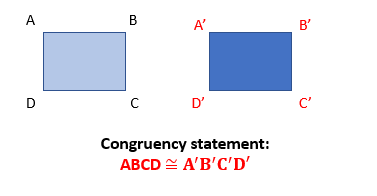## Rotation

• In rotation, a figure or image is turned around a center in a specific direction through a given angle.
• A rotation needs
1. a center of rotation (a fixed point)
2. degree of rotation (for example, 90o or 180o)
3. a direction (clockwise or anticlockwise)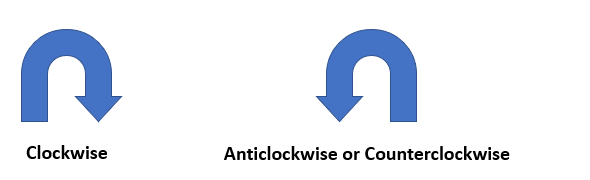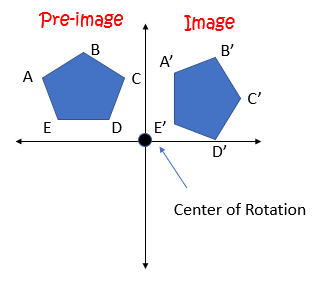## Solved Examples

Question 1: If point $P\left(1,-6\right)$ is rotated $180°$ counter-clockwise about the origin, point ${P}^{\text{'}}$ is obtained. What are the coordinates of point ${P}^{\text{'}}$?

Solution: If a point $P\left(x,y\right)$ is rotated $180°$ counter-clockwise, then the coordinates of the image point is ${P}^{\text{'}}\left(-x,-y\right)$.

So, the coordinates of the image point of $P\left(1,-6\right)$ will be ${P}^{\text{'}}\left(-1,6\right)$.

Question 2: If point $P\left(-12,5\right)$ is rotated $90°$ counter-clockwise about the origin, point ${P}^{\text{'}}$ is obtained. What are the coordinates of point ${P}^{\text{'}}$?

Solution: If a point $P\left(x,y\right)$ is rotated $90°$ counter-clockwise, then the coordinates of the image point is ${P}^{\text{'}}\left(-y,x\right)$.

So, the coordinates of the image point of $P\left(-12,5\right)$ will be ${P}^{\text{'}}\left(-5,-12\right)$.

Question 3: An equilateral triangle PQR as shown below is rotated $270°$ clockwise. What are the coordinates of the image of vertex P?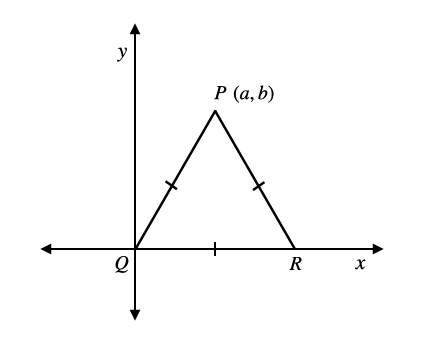Solution: A rotation of $270°$ in clockwise sense is equivalent to a rotation of $90°$ in counter-clockwise sense.

If a point $P\left(x,y\right)$ is rotated $90°$ counter-clockwise, then the coordinates of the image point is ${P}^{\text{'}}\left(-y,x\right)$.

So, the coordinates of the image point of $P\left(a,b\right)$ will be ${P}^{\text{'}}\left(-b,a\right)$.

## Cheat Sheet

The following table summarizes the ordered pairs before and after the described rotation.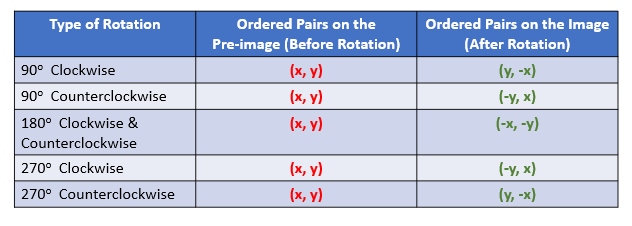## Blunder Areas

• When a figure rotates from one quadrant to another quadrant, the figure is rotated at an increment of 90o clockwise or counter-clockwise.
• Unless noted otherwise, the angle of rotation is always counterclockwise.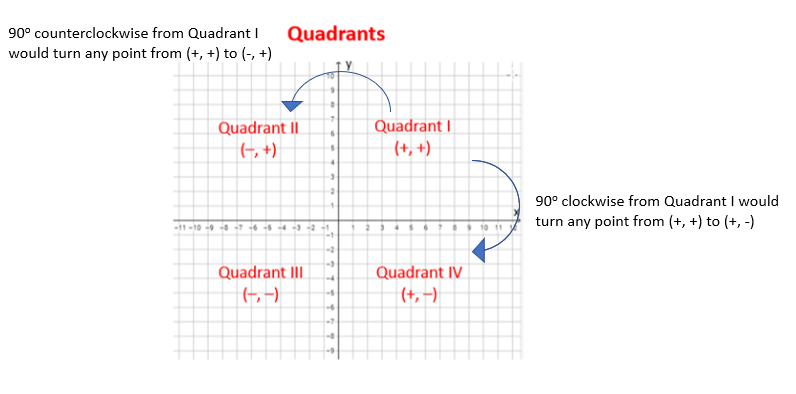• Pre-image (before rotation) and image (after rotation) are congruent (same size and shape).